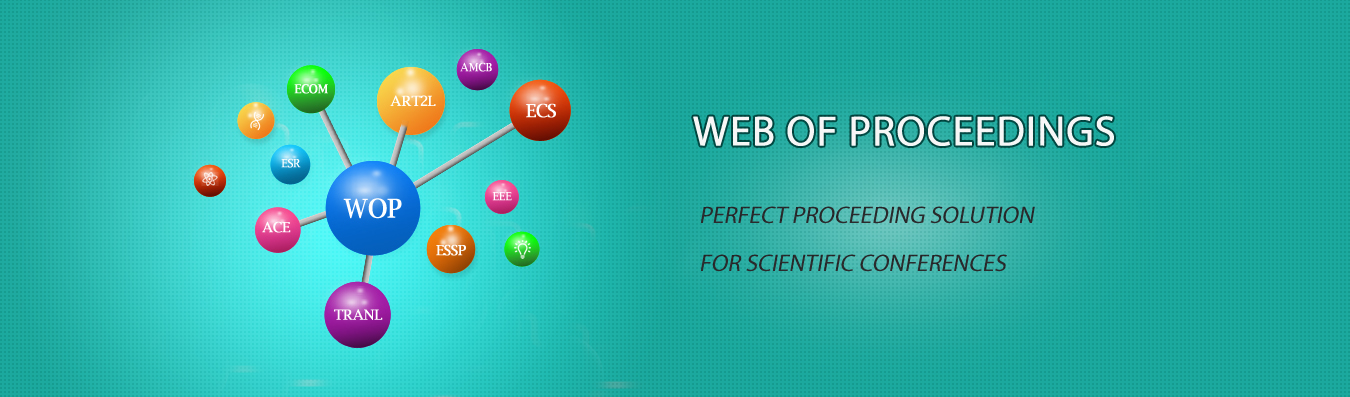The best way to conference proceedings by Francis Academic Press### Research on Structure Learning Theory and Algorithm Based on Probabilistic Graphical Model

DOI: 10.25236/iceeecs.2018.062

Li Feng

Li Feng

#### Abstract

Probabilistic graphical model can effectively deal with uncertainty reasoning, and learning the probabilistic graphical model from the sample data is an important problem in practical application. The representation of the probabilistic graphical model consists of two parts, parameters and structure. The learning algorithm is also divided into parameter learning and structure learning. In this paper, the structure learning algorithms based on probabilistic graphical model network are introduced in detail. The structural learning algorithms are also summarized based on the differences in the characteristics of structural learning algorithms, and the algorithm of Markov network structure learning is summarized. Finally, this paper points out the open problems of the probabilistic graphical model learning and its further research directions.

#### Keywords

Probabilistic graphical model, Bayesian network, Markov network.